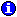﻿ <<*If*>> Prompt
Pathagoras Help System

<<*If*>> Prompt

 <<*If*>> Prompt<<*If*>> PromptWhile <<*Ask*>> prompts will constitute the bulk of the document logic table, it is the <<*IF*>> prompt that forms the backbone of document logic process. You will need the <<*IF*>> prompt to declare your comparisons and to set up your decision and branching points.

Let's say you are drafting a document that has optional text dealing with children. You want the text to be kept in the document when there are children and discarded when there are none. Let's further say that the document contains other language that you want to keep when there are minor children, but which should be discarded where there are none.

Using AskOptional prompts, you might ask the question "Are there children?". If (but only if) that question is answered "Yes", you might then ask "Are there minor children?". (Of course, if there were no children, there couldn't be  any minor children; therefore the question should not be asked..)

Here is the way this sequence might appear:NOTE: The values "Yes", "True" and "1" are functional equivalents. The above could have been written: <<*AskOptional*!Children!Are there children?*>> <<*If*!Children!="1",<<*AskOptional*!Minors!Are there minor children*>>,!minors!="False">>

Let's study the structure.

1. Note first that a !groupname! (in this case !children!) is used. As with other !group!, the name ties the various elements of the document together.

2. Now note the classic three-part "if . . . then" programming structure in line 2:

"If Condition, (If 'True' Action), (If 'False' Action)"

This three-part logic structure is practically universal. Simple commas are used to separate the three elements.

As applied to the example, the user first encounters the initial (Children) question. The user provides either a "True" or "False" (or 'Yes' or 'No') answer. The "If" prompt is encountered immediately after. Pathagoras 'measures' the value of the !Children! group, and responds accordingly. If !Children! was answered "Yes" (by checking the checkbox), Pathagoras presents the prompt that follows the first comma. If Pathagoras sees that !Children! was not answered "Yes", it processes the information that follows the second comma.  portion is triggered by the

The sequence of the Ask prompts is, of course, critical. So is the proper spelling of the !GroupNames!. However, "True" and "Yes" are totally interchangeable, as are "False" and "No".

See Bigger Example for more examples of the <<*If*>> prompt.

A bit of Math:

Pathagorsa can do a bit of math for you if you desire. When a !GroupValue! has been set to a numerical amount, either through an <<*AskRepeat*>> or a <<*Set*>> function, you can perform a math function on the values. If the value matches the comparison set up, then the 'True' part of the <<*If*>> is processed, otherwise, the 'False' part is.

<<*If*!NumCdn!+!NumPets!>5,!CrazyHouse!=”True”,!CrazyHouse!=”False”)>>